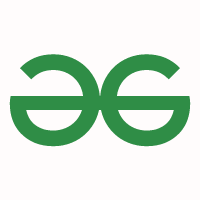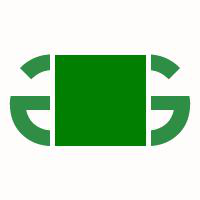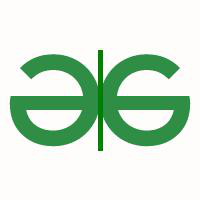# Python Pillow – ImageDraw Module

• Last Updated : 18 Jul, 2021

Python’s Pillow which is a fork of the discontinued Python Imaging Library (PIL) is a powerful library that is capable of adding image processing capabilities to your python code. Pillow offers many modules that ease the process of working and modifying images.

In this article, we will have a look at the ImageDraw module of this library. ImageDraw provides a variety of methods to, as its name suggests, draw on images. With the help of this module, we can draw lines, circles, rectangles and, even write and format text on an image.

Attention geek! Strengthen your foundations with the Python Programming Foundation Course and learn the basics.

To begin with, your interview preparations Enhance your Data Structures concepts with the Python DS Course. And to begin with your Machine Learning Journey, join the Machine Learning - Basic Level Course

## Drawing common shapes on image

The image we will be using can be displayed using PIL as follows:

## Python

 `# Importing Image and ImageDraw from PIL``from` `PIL ``import` `Image, ImageDraw`` ` `# Opening the image to``# be used and displaying it``img ``=` `Image.``open``(``'img_path.png'``)``img.show()`

OutputThe output image is as follows

We can draw shapes and figures on an Image using the Draw method by firstly creating a Draw object.

### Drawing a rectangle on the image:

For drawing a rectangle, we use the rectangle drawing method of the ImageDraw module:

Syntax: ImageDraw.rectangle(xy, fill, outline, width)

The paramaters for this method are:

• xy : Corresponds to the tuple of set of points in the upper left corner and lower right coordinates enclosing your shape. The points are passed in a tuple as follows :  (upper left x-coordinate, upper left y-coordinate, lower right x-coordinate, lower right y-coordinate)
• fill : Corresponds to the tuple of RGB colour values to fill the shape with.
• outline : Corresponds to the tuple of RGB colour values assigned for the shape’s boundary.
• width : Integer value corresponding to the thickness of the boundary of the shape.NOTE: The parameters are similar across various shape drawing methods.

## Python

 `# Importing Image and ImageDraw from PIL``from` `PIL ``import` `Image, ImageDraw`` ` `# Opening the image to be used``img ``=` `Image.``open``(``'img_path.png'``)`` ` `# Creating a Draw object``draw ``=` `ImageDraw.Draw(img)`` ` `# Drawing a green rectangle``# in the middle of the image``draw.rectangle(xy ``=` `(``50``, ``50``, ``150``, ``150``),``               ``fill ``=` `(``0``, ``127``, ``0``),``               ``outline ``=` `(``255``, ``255``, ``255``),``               ``width ``=` `5``)`` ` `# Method to display the modified image``img.show()`

Output:Output image of the rectangle method

### Drawing an ellipse(circle) on the image:

For drawing an ellipse shape, we use the ellipse method of the ImageDraw methods:

Syntax: ImageDraw.ellipse(xy, fill, outline, width)

The co-ordinates you will provide in xy will act as a box in which the circle will be enclosed.

## Python

 `# Importing Image and ImageDraw from PIL``from` `PIL ``import` `Image, ImageDraw`` ` `# Opening the image to be used``img ``=` `Image.``open``(``'img_path.png'``)`` ` `# Creating a Draw object``draw ``=` `ImageDraw.Draw(img)`` ` `# Drawing a green circle on the image``draw.circle(xy ``=` `(``50``, ``50``, ``150``, ``150``), ``            ``fill ``=` `(``0``, ``127``, ``0``),``            ``outline ``=` `(``255``, ``255``, ``255``),``            ``width ``=` `5``)`` ` `# Method to display the modified image``img.show()`

Output:Output image of the ellipse method

### Drawing a line on the image:

For drawing a line, we use the line method of the ImageDraw methods:

Syntax: lImageDraw.ine(xy, fill, width)

Here, the outline parameter is not considered and the width will determine how long the line should be.

## Python

 `# Importing Image and ImageDraw from PIL``from` `PIL ``import` `Image, ImageDraw`` ` `# Opening the image to be used``img ``=` `Image.``open``(``'img_path.png'``)`` ` `# Creating a Draw object``draw ``=` `ImageDraw.Draw(img)`` ` `# Drawing a green vertical``# line in the middle image``draw.line(xy``=``(``50``, ``150``, ``150``, ``50``),``          ``fill``=``(``0``, ``128``, ``0``), width ``=` `5``)`` ` `# Method to display the modified image``img.show()`

Output:Output image of the line method

### Drawing a polygon on the image:

We can draw a polygon of the desired shape by using the polygon method of the ImageDraw methods:

Syntax: ImageDraw.polygon(xy, fill, outline)

The xy tuple parameter will contain co-ordinates based on the number of sides you want for your shape. Here, the width parameter is not valid.

## Python

 `# Importing Image and ImageDraw from PIL``from` `PIL ``import` `Image, ImageDraw`` ` `# Opening the image to be used``img ``=` `Image.``open``(``'img_path.png'``)`` ` `# Creating a Draw object``draw ``=` `ImageDraw.Draw(img)`` ` `# Drawing a green diamond-shaped``# polygon in the middle of the image``draw.polygon(xy``=``(``50``, ``150``, ``150``, ``50``),``             ``fill``=``(``0``, ``128``, ``0``), ``             ``outline``=``(``255``, ``255``, ``255``))`` ` `# Method to display the modified image``img.show()`

Output:Output image of the polygon method

Similarly, we can draw some other shapes using these methods:

1. Arc: ImageDraw.arc(xy, start, end, fill, width)
2. Chord (Bow-shape): ImageDraw.chord(xy, start, end, fill, outline, width)
3. Point: ImageDraw.point(xy, fill)
4. Pieslice: ImageDraw.pieslice(xy, start, end, fill, outline, width)

The start and end parameters correspond to the degree of angles in clockwise direction, which will be connected with a line.

## Writing text on image:

Using our Draw object we can also write text on an image. It can be done using the Text method:

Syntax: ImageDraw.text(xy, text, fill, font, anchor, spacing, align, direction, features, language, stroke_width, stroke_fill, embedded_color)

We will also be using ImageFont from PIL to use the desired font for our text.

## Python

 `# Importing Image, ImageDraw and ImageFont ``# from PIL``from` `PIL ``import` `Image, ImageDraw, ImageFont`` ` `# Opening the image to be used``img ``=` `Image.``open``(``'img_path.png'``)`` ` `# Creating an instance for``# the font to be used using ImageFont``# Here we pass the font name and``# the font size as arguments``fnt ``=` `ImageFont.truetype(``"Pillow/Tests/fonts/FreeMono.ttf"``, ``20``)`` ` `# Creating a Draw object``draw ``=` `ImageDraw.Draw(img)`` ` `# Drawing the text on the image``draw.text(xy``=``(``25``, ``160``),``          ``text``=``"Hello, Geeks!"``,``          ``font``=``fnt,``          ``fill``=``(``0``, ``127``, ``0``))`` ` `img.show()`

Output:Output image of the text method

My Personal Notes arrow_drop_up